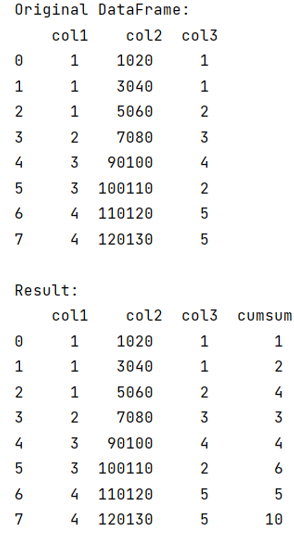# How to Calculate Cumulative Sum by Group (cumsum) in Pandas?

Given a pandas dataframe, we have to calculate cumulative sum by Group (cumsum).
Submitted by Pranit Sharma, on September 13, 2022

Pandas is a special tool that allows us to perform complex manipulations of data effectively and efficiently. Inside pandas, we mostly deal with a dataset in the form of DataFrame. DataFrames are 2-dimensional data structures in pandas. DataFrames consist of rows, columns, and data.

## Calculating Cumulative Sum by Group (cumsum) in Pandas

Suppose, we have a DataFrame with multiple columns and we need to groupby some columns, and then we need to find the cumulative sum (cumsum) within a group.

For this purpose, we will first perform groupby() on column/columns and then we will use the transform() method to pass the cumsum method inside it.

Whenever we want to perform some operation on the entire DataFrame we use the transform method. The transform() method passes a single column of a group at a time in the form of a series inside the function which is described in the transform() method.

The function which is described inside the transform() method must return a sequence of the same length as the group.

Let us understand with the help of an example,

## Python code to calculate cumulative sum by group (cumsum) in Pandas

```# Importing pandas package
import pandas as pd

# Creating a dictionary
d = {
'col1':[1,1,1,2,3,3,4,4],
'col2':[1020,3040,5060,7080,90100,100110,110120,120130],
'col3':[1,1,2,3,4,2,5,5]
}

# Creating a DataFrame
df = pd.DataFrame(d)

# Display original DataFrame
print("Original DataFrame:\n",df,"\n")

# Getting group values and converting to dictionary
df['cumsum'] = df.groupby('col1')['col3'].transform(pd.Series.cumsum)

# Display result
print("Result:\n",df)
```

Output:What's New (MCQs)

Top Interview Coding Problems/Challenges!

Languages: » C » C++ » C++ STL » Java » Data Structure » C#.Net » Android » Kotlin » SQL
Web Technologies: » PHP » Python » JavaScript » CSS » Ajax » Node.js » Web programming/HTML
Solved programs: » C » C++ » DS » Java » C#
Aptitude que. & ans.: » C » C++ » Java » DBMS
Interview que. & ans.: » C » Embedded C » Java » SEO » HR
CS Subjects: » CS Basics » O.S. » Networks » DBMS » Embedded Systems » Cloud Computing
» Machine learning » CS Organizations » Linux » DOS
More: » Articles » Puzzles » News/Updates

© https://www.includehelp.com some rights reserved.